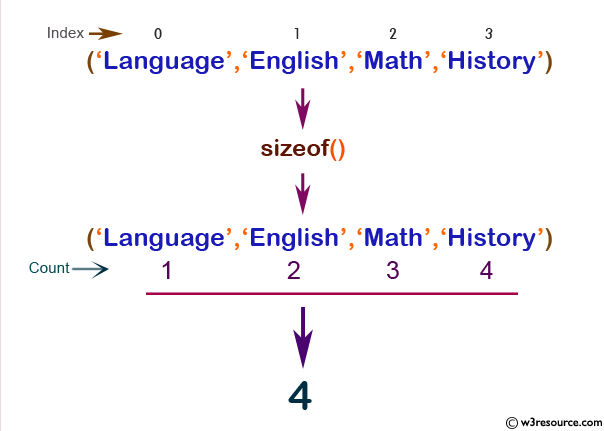# PHP: sizeof() function

## PHP: Count all elements in an array

The sizeof() function is used to count the elements of an array or the properties of an object. This function is an alias of count().

Version:

(PHP 4 and above)

Syntax:

`sizeof(array_name, mode) `

Parameters:

Name Description Required /
Optional
Type
array_name Specifies the array or object to count. Required Array
mode Sets the mode of the function.
Possible values :
COUNT_RECURSIVE (or 1) Here the count() function counts the array recursively. This is useful for counting all the elements of a multidimensional array.
The default value is 0.
Optional Integer

Return value:

The number of elements in array_name.

Value Type: Array.

Example:

``````<?php
\$a = 'Language';
\$a = 'English';
\$a = 'Math';
\$a = 'Science';
\$result = sizeof(\$a);
echo \$result;
?>
``````

Output:

`4`

Pictorial Presentation:View the example in the browser

Practice here online :

PHP Function Reference

Previous: shuffle
Next: sort

﻿

## PHP: Tips of the Day

PHP: Extract numbers from a string

```\$str = 'In My Cart : 11 12 items';
preg_match_all('!\d+!', \$str, \$matches);
print_r(\$matches);
```